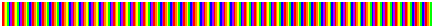# prism

Prism colormap array

•## Syntax

``c = prism``
``c = prism(m)``

## Description

````c = prism` returns the prism colormap as a three-column array with the same number of rows as the colormap for the current figure (`gcf`). If no figure exists, then the number of rows is equal to the default length of 256. Each row in the array contains the red, green, and blue intensities for a specific color. The intensities are in the range [0,1], and the color scheme is a repeated sequence of the rainbow colors.```

example

````c = prism(m)` returns the colormap with `m` colors.```

## Examples

collapse all

Create a scatter plot with the default colors.

```x = [3.5 3.3 5 6.1 4 2]; y = [14 5.7 12 6 8 9]; sz = 100*[6 100 20 3 15 20]; c = [1 2 3 4 5 6]; scatter(x,y,sz,c,'filled','MarkerEdgeColor','k','MarkerFaceAlpha',.5); xlim([1 7]); ylim([1 16]);```Get the prism colormap array with six entries. Then replace the colormap in the scatter plot.

```c = prism(6); colormap(c);```## Input Arguments

collapse all

Number of colors, specified as a nonnegative integer. The default value of `m` is the number of colors in the current figure's colormap. If no figure exists, the default value is `256`.

Data Types: `single` | `double`

## Version History

Introduced before R2006a

expand all